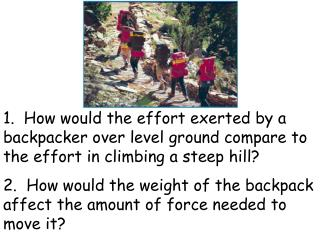# 1. How would the effort exerted by a backpacker over level ground compare to the effort in climbing a steep hill? - PowerPoint PPT PresentationDownload Presentation1. How would the effort exerted by a backpacker over level ground compare to the effort in climbing a steep hill?

1. How would the effort exerted by a backpacker over level ground compare to the effort in climbing a steep hill?
Download Presentation## 1. How would the effort exerted by a backpacker over level ground compare to the effort in climbing a steep hill?

- - - - - - - - - - - - - - - - - - - - - - - - - - - E N D - - - - - - - - - - - - - - - - - - - - - - - - - - -
##### Presentation Transcript

1. 1. How would the effort exerted by a backpacker over level ground compare to the effort in climbing a steep hill? 2. How would the weight of the backpack affect the amount of force needed to move it?

2. Work and Machines A. Work 1. The transfer of energy when a force makes an object move. 2. W = f ·d (Unit is a joule or N ·m) Box being given energy 3. Energy is transferred between objects when work is done.

3. B. Power 1. The rate at which work is done. 2. P = W / t 3. Unit is the watt (1 joule / second) 4. Btu = 1,055 watts (for heating and cooling units) 5. Horsepower = 746 watts (for motors and engines)

4. C. Using Machines 1. A machine makes doing work easier. 2. They may multiply the applied force. The car may weigh a lot, but you don’t have to use nearly that much force to lift it with a jack.

5. 3. They may increase the distance over which a force is applied. In this case, the amount of force necessary to push the chair up the ramp was decreased.

6. 4. They may change the direction a force has to be applied. The nail comes up as the person pulls to the side.

7. D. Important terms for machines 1. Resistance – the force being moved (FR) 2. Effort – the force being used to move a resistance (FE) 3. Effort Distance – the distance the effort force moves through (de) 4. Resistance Distance – how far the resistance moves (dr)

8. E. Work Calculations 1. Work Input – the amount of work done on a simple machine. 2. Win = FE • de 3. Work output – the amount of work the machine actually does. 4. Wout = FR • dr

9. F. Conservation of Energy 1. No machine can create energy, so Wout can never be greater than Win 2. In reality, Wout is alwaysless than Win because of friction producing heat; the heat had to come from the energy put into the machine. 3. An ideal machine is theoretical; it does not take friction into account. 4. Ideal machine: Win = Wout

10. G. Mechanical Advantage 1. MA is the number of times a machine multiplies the effort force. 2. MA = FR / FE & MA = de / dr H. Efficiency 1. Measures how much of the work input is changed into useful output 2. Efficiency = (Wout / Win) x 100%

11. 3. Lubricants (such as oiland graphite) reduce friction & increase efficiency. Oil fills the space between surfaces so high spots don’t rub against each other.

12. I. The Simple Machines 1. Levers a. 1st class: b. 2nd class: c. 3rd class: d. IMA = effort arm / resistance arm FE FR FE FR FR FE

13. 2. Pulleys a. Fixed pulley 1) changes only the direction of a force 2) always has IMA = 1 b. Movable pulley 1) attached to object 2) IMA = 2

14. c. Block and Tackle This strand does not count toward the IMA 1) system of fixed and movable pulleys 2) IMA = number of strands supporting the resistance

15. 3. Wheel and Axle a. IMA = rw / ra b. Gears are modified forms of the wheel and axle

16. 4. Inclined Planes: IMA = Ls / Lh 5. The Screw a. Modified inclined plane wrapped around a cylinder b. The pitch of the threading determines the IMA 6. The Wedge: two inclined planes back-to-back